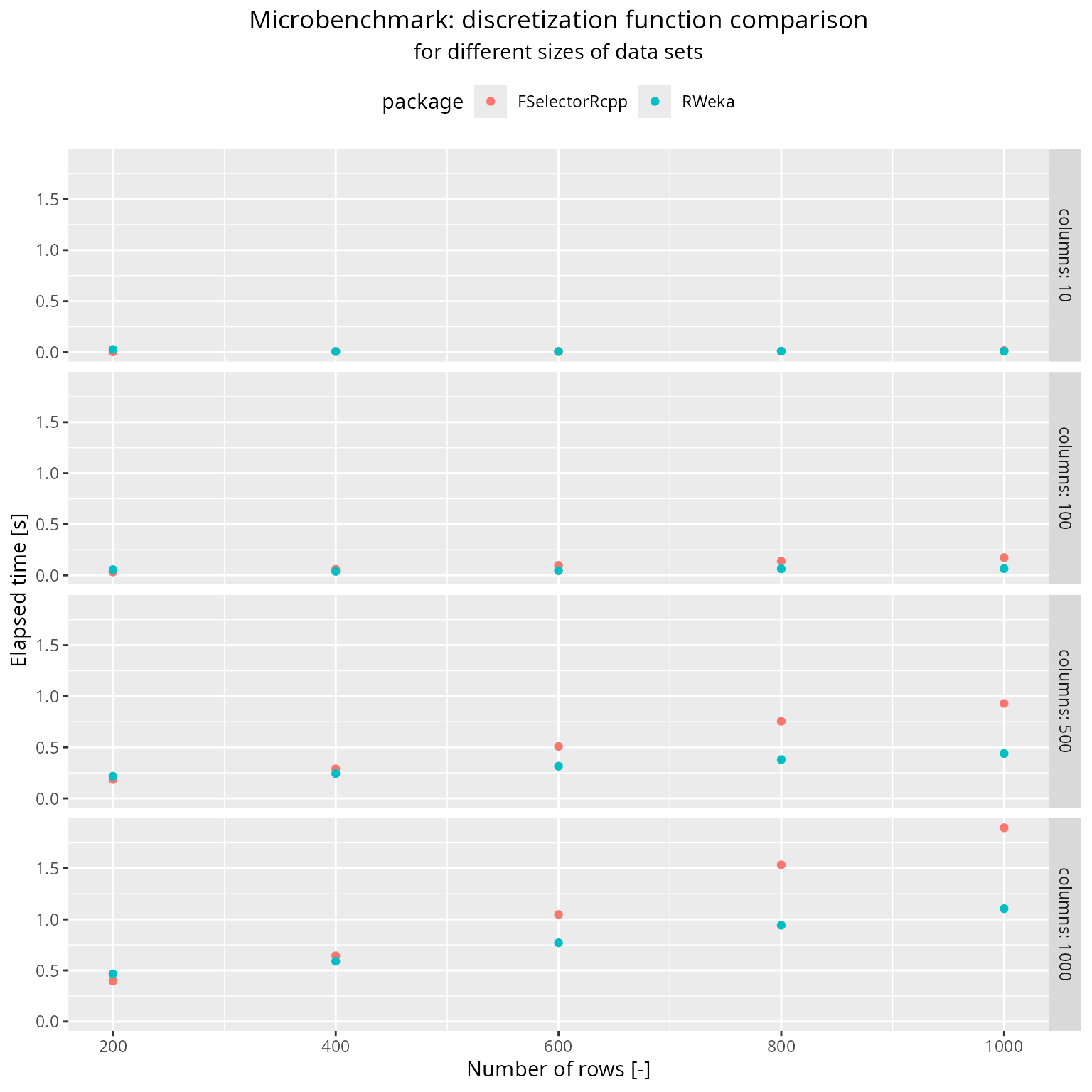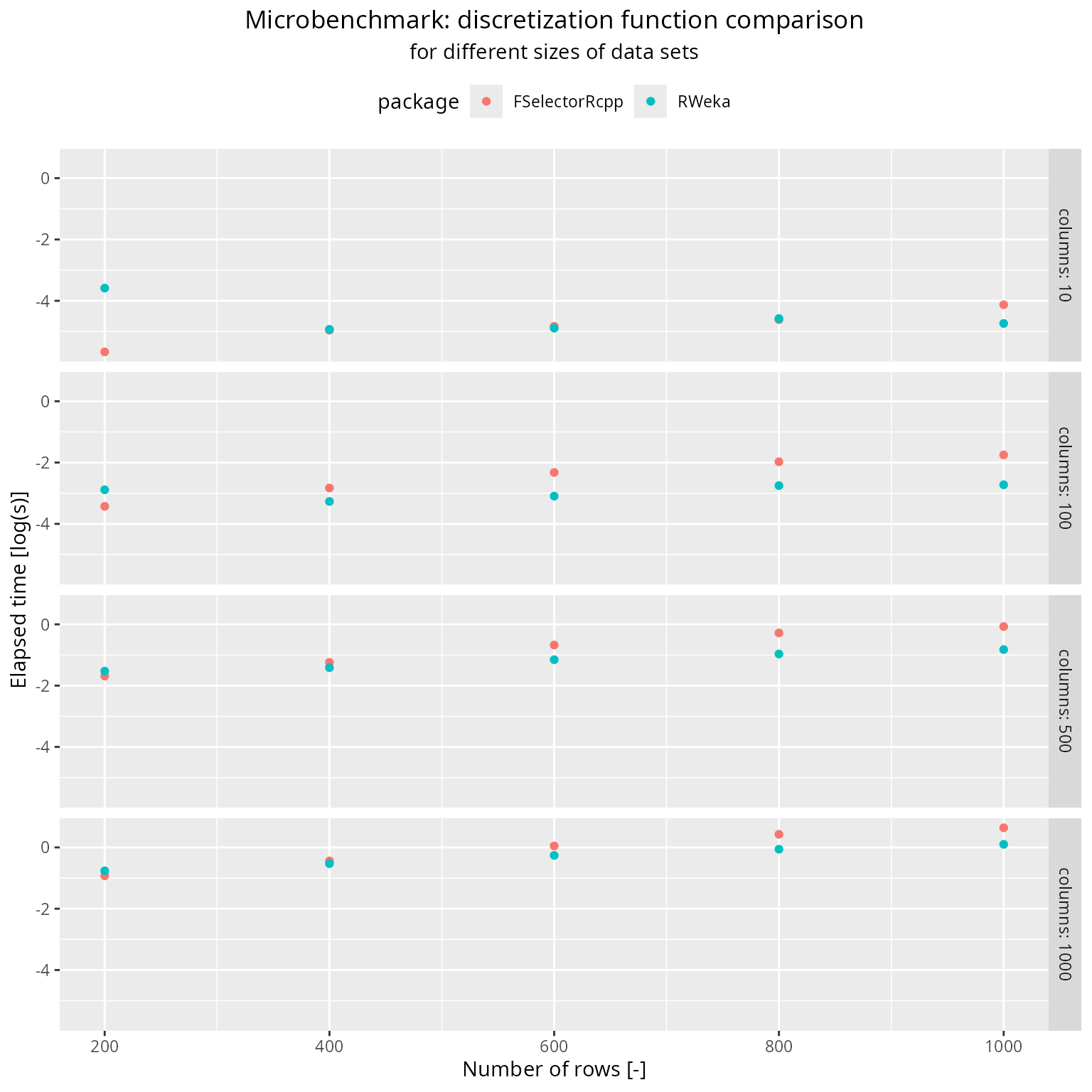library(microbenchmark)
library(FSelectorRcpp)
library(RWeka)

In the statistical modelling, the discretization is the process of transferring continuous explanatory variables into discrete counterparts. Problems caused by categorization of continuous variables are known and widely spread (Harrell 2015), but in some cases there appear an algorithmic requirement for the discretization. Moreover, there exist few algorithms, like Decision Trees (Salzberg 1994), where continuous attributes are discretized during the learning process. Other reason for variable discretization include increasing the speed of induction algorithms (Catlett 1991).

Even though many categorization algorithms have been developed (Holte 1993, @Chan169942), in this article we focus on a recursive entropy minimization heuristic for categorization coupled with a Minimum Description Length (MDL) critetion (Rissanen 1986) that controls the number of intervals produced over the continuous space. This is motivated by the original usage of this algorithm in FSelector package, which is our baseline.

(Dougherty, Kohavi, and Sahami 1995) showed better performance of classification for discretized feature set on real-world datasets and states that the described method was found promising by the authors not only for the local discretization but also for global discretization (Ting 1994).

Comparisons on RTCGA data

The Cancer Genome Atlas (TCGA) is a comprehensive and coordinated effort to accelerate our understanding of the molecular basis of cancer through the application of genome analysis technologies, including large-scale genome sequencing - http://cancergenome.nih.gov/.

RTCGA team converted selected datasets from this study into few separate packages that are hosted on Bioconductor. These R packages make selected datasets easier to access and manage. Data sets in RTCGA packages are large and cover complex relations between clinical outcomes and genetic background.

To use RTCGA install package with instructions from it’s Bioconductor home page

## try http:// if https:// URLs are not supported
source("https://bioconductor.org/biocLite.R")
biocLite("RTCGA")

Dependent variable

Specifies whether a sample came from tumour or healthy tissue. It is denoted by 14th sign in bcr_patiend_barcode variable in BRCA.rnaseq dataset that containt RNA-Seq genes’ expressions for patients suffering from Breast Cancer.

Independent variables

It is mandatory to get rid of special signs from their names.

library(RTCGA.rnaseq)
BRCA.rnaseq <- RTCGA.rnaseq::BRCA.rnaseq
BRCA.rnaseq$bcr_patient_barcode <- factor(substr(BRCA.rnaseq$bcr_patient_barcode, 14, 14))
names(BRCA.rnaseq) <- gsub(pattern = "?", replacement = "q",
x = names(BRCA.rnaseq), fixed = TRUE)
names(BRCA.rnaseq) <- gsub(pattern = "[[:punct:]]", replacement = "_",
x = names(BRCA.rnaseq))

Discretization Benchmarks

library(ggplot2)
library(pbapply)
library(tibble)
names_by <- function(nameBy, vecBy) {
c(paste0(nameBy,
sapply(max(nchar(vecBy)) - nchar(vecBy),
function(zeros) {
paste0(rep(0, zeros), collapse = "")
}),
vecBy))
}

get_times <- function(fun, vecRows, vecCols, nTimes) {
forRows <- pblapply(vecRows, function(nRows) {
forCols <- pblapply(vecCols + 1, function(nCols) {
suppressWarnings(microbenchmark(fun(bcr_patient_barcode ~ .,
BRCA.rnaseq[1:nRows, 1:nCols]),
times = nTimes))
})
colsNames <- names_by(nameBy = "columns_", vecBy = vecCols)
setNames(forCols, colsNames)
})
rowsNames <- names_by(nameBy = "rows_", vecBy = vecRows)
setNames(forRows, rowsNames)
}

make_df <- function(bm_data, package) {
data_frame(
rows = rep(
x = as.integer(
gsub(
pattern = "rows_0*",
replacement = "",
x = names(bm_data)
)
),
each =  unique(sapply(bm_data, length))
),
columns = as.integer(
gsub(
pattern = "columns_0*",
replacement = "",
x = as.vector(sapply(bm_data, names))
)
),
time = as.vector(
sapply(
bm_data,
function(setOfCols) {
sapply(
setOfCols,
function(set) {
median(set\$time) / 10 ^ 9
}
)
}
)
),
package = package
)
}

bm_fsr_discretize <- get_times(fun = discretize,
vecRows = seq(from = 200, to = 1000, by = 200),
vecCols = c(10, 100, 500, 1000),
nTimes = 1L)
bm_rwk_discretize <- get_times(fun = Discretize,
vecRows = seq(from = 200, to = 1000, by = 200),
vecCols = c(10, 100, 500, 1000),
nTimes = 1L)

df_fsr <- make_df(bm_data = bm_fsr_discretize, package = "FSelectorRcpp")
df_rwk <- make_df(bm_data = bm_rwk_discretize, package = "RWeka")
bm_df <- rbind(df_fsr, df_rwk)

make_plot <- function(y) {
yUnit <- gsub(pattern = "time",
replacement = "s",
x = y)

ggplot(data = bm_df, aes_string(x = "rows", y = y, color = "package")) +
facet_grid(columns ~ ., labeller = label_both) +
geom_point() +
xlab("Number of rows [-]") +
ylab(paste0("Elapsed time [", yUnit, "]")) +
ggtitle(label = "Microbenchmark: discretization function comparison",
subtitle = "for different sizes of data sets") +
theme(plot.title = element_text(hjust = 0.5),
plot.subtitle = element_text(hjust = 0.5),
legend.position = "top")
}

bm_plot <- make_plot("time")
bm_plot_log <- make_plot("log(time)")

Plots